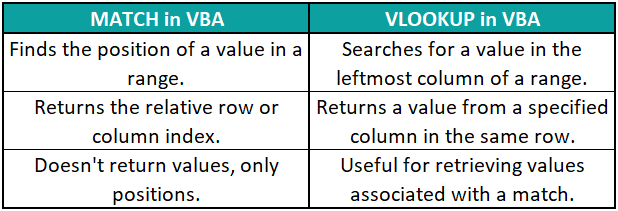# Vlookup in VBA ExcelArticle byExcelMojo Team## What is Excel VBA VLOOKUP Function?

In Excel VBA, the VLOOKUP function retrieves data from a table or range based on a specific lookup value. VLOOKUP stands for “Vertical Lookup,” a widely used function to perform vertical searches in a worksheet. It’s commonly used to search for a value in the leftmost column of a table and return a corresponding value from a specified column.

Let us know more about the syntax of the VLOOKUP function in Excel VBA.

Application.WorksheetFunction.VLookup(lookup_value, table_array, col_index, [range_lookup])

Where:

• lookup_value: The value you want to look up in the first column of the table_array.
• table_array: The range of cells that contains the data you want to search through. This should include both the lookup column and the columns from which you want to retrieve data.
• col_index: The relative column index in the table_array from which you want to retrieve data. The leftmost column in the table_array is column 1.
• range_lookup: Optional parameter. If set to True (or omitted), VLOOKUP will perform an approximate match. If set to False, it will perform an exact match.

Consider the following example where we get the type of product “Apple” by performing the VLOOKUP in VBA Excel function in the table given below:

It will result in the output:

###### Key Takeaways
• VLOOKUP in VBA searches for a value in the leftmost column of a range. It retrieves a corresponding value from a specified column in the same row.
• It is useful for performing lookups and obtaining the associated data. VLOOKUP requires exact matching by default.
• When using VLOOKUP in VBA Excel, the data should be sorted for accurate results. It tends to be slower with large datasets.
• VLOOKUP in VBA is limited to a one-column lookup. It requires specifying the lookup value and range.

### How to Use VLookup in Excel VBA?

To perform the VLOOKUP function in Excel VBA, go through the following steps below.

1. Go to the “Developer” section in the toolbar and click the “Visual Basic” option. Now, the VBA Editor opens to add functions and Sub procedures. Then, click the “Insert” and “Module” buttons to create a new module or blank page.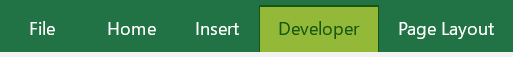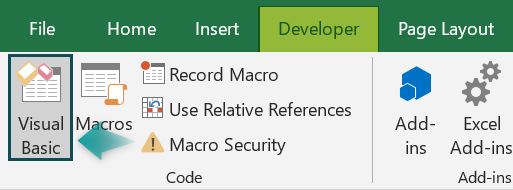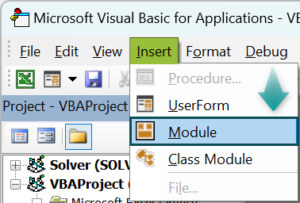2. Initialize a sub-procedure to get the salary of a given employee from the given table.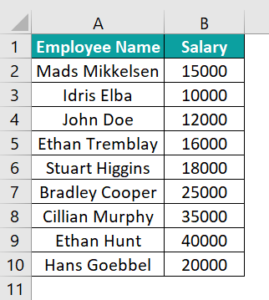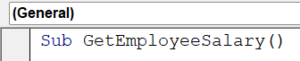3. Initialize a string variable to store the name of the employee to be searched and a range variable to store the range of the table, the salary column in the table and the salary value to print as output.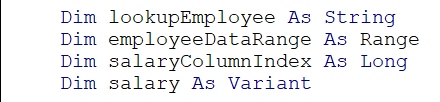4. Get the value of the employee to be searched.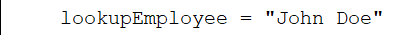5. Set the range of the table.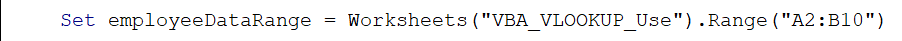6. Get the column index of the salary column.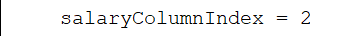7. Get the salary of the employee using the VLOOKUP in VBA Excel function.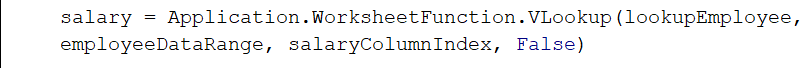Code:

Sub GetEmployeeSalary()
Dim lookupEmployee As String
Dim employeeDataRange As Range
Dim salaryColumnIndex As Long
Dim salary As Variant
lookupEmployee = “John Doe”
Set employeeDataRange = Worksheets(“VBA_VLOOKUP_Use”).Range(“A2:B10”)
salaryColumnIndex = 2
salary = Application.WorksheetFunction.VLookup(lookupEmployee, employeeDataRange, salaryColumnIndex, False)
If Not IsError(salary) Then
MsgBox “Salary of ” & lookupEmployee & ” is: \$” & salary
Else
End If
End Sub

8. Run the code to find the salary of “John Doe”.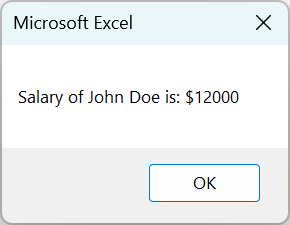Now that we know how to implement VLOOKUP in VBA Excel, let us go through some examples.

### Examples

Let us look at some examples of how to implement the VLOOKUP in VBA.

#### Example #1

A company has an employee database recording the distance between each employee’s residence and the office. The company wants to implement a policy where they randomly search for the employee’s name and will call them to work on a Saturday based on their distance in miles. It can be done using VLOOKUP in Excel VBA. Let us see how to do so by referring to the table below:

• Step 1: Start a subroutine to calculate the employee distance.
• Step 2: Initialize variables to accept the input name, the value of the distance and the range/ size of the table.
• Step 3: Set the sheet number in which this subroutine will work.
• Step 4: Get the input from the user.
• Step 5: Set the range/size of the table.
• Step 6: Find the value in the table using the VLOOKUP function and return the value from column 4, the column number of distance and then print the space.

Code:

Sub CalculateEmployeeDistance()
Dim EmpName As String
Dim distance As Long
Dim rng As Range
Set empData = Worksheets(“Example_1”)
EmpName = InputBox(“Enter a name: “)
Set rng = Range(“A2:D9”)
dist = Application.WorksheetFunction.VLookup(EmpName, rng, 4, False)
Debug.Print “Name: ” & EmpName & “, Distance: ” & dist & “km”
End Sub

• Step 7: Run the code implementing the VBA VLOOKUP function.

After this, the output will be printed in the immediate tab.

#### Example #2

Suppose a shop has a lists catalogue and they have sold the following products in the table below. They need to dynamically update the revenue obtained by selling these items. It can be done using the VLOOKUP function in Excel VBA.

• Step 1: Create a subroutine to calculate the total revenue.
• Step 2: Define two variables rng to define the range of the table, and cell as an iterative integer to be used in a FOR loop.
• Step 3: Define multiple variables in this subroutine, such as a Worksheet variable, the size of the sales table and the size of the product table, an iterative variable, product names, the number of products sold, the price of the product, and the total revenue.
• Step 4: Define the worksheet in which this subroutine will work.
• Step 5: Define the range of the sales table and the product table.

The xlUp is used to imitate the up-arrow key. It is used to check the last non-empty cell in column “A” and returns the size of the rows till the last non-empty row.

• Step 6: Initialize a FOR loop to run through the sales table and define the columns for the product name and quantity sold.
• Step 7: Find the cost of each product by comparing the values of both tables using the VLOOKUP function.

Here, we can see that the VLOOKUP function can also be used to compare the leftmost value between two tables.

• Step 8: Get the total sales revenue by multiplying the quantity and product price. Fill in the values in the third column.

After all the values are parsed through the for loop, it exits automatically and exits the subroutine.

Code:

Sub CalculateTotalRevenue()
Dim salesData As Worksheet
Dim lastRowSales As Long
Dim lastRowProducts As Long
Dim i As Long
Dim productName As String
Dim quantitySold As Double
Dim productPrice As Double
Dim totalRevenue As Double
Set salesData = Worksheets(“Example_2”)
‘define the two tables
lastRowSales = salesData.Cells(salesData.Rows.Count, “A”).End(xlUp).Row
lastRowProducts = salesData.Cells(salesData.Rows.Count, “G”).End(xlUp).Row
For i = 2 To lastRowSales
productName = salesData.Cells(i, 1).Value
quantitySold = salesData.Cells(i, 2).Value
productPrice = Application.WorksheetFunction.VLookup(productName, salesData.Range(“G2:H” & lastRowProducts), 2, False)
totalRevenue = quantitySold * productPrice
salesData.Cells(i, 3).Value = totalRevenue
Next i
End Sub

• Step 9: Run the code for the above table. The output is shown below:

#### Example #3

To prepare a student report, a college has a list of students studying in various departments whose Grades are displayed. We need to print the average value of all the students to add it to the report card. It can be done using the VBA VLOOKUP function.

Here, we need to print the average grades for each student.

• Step 1: Initialize a sub-procedure to print the average.
• Step 2: Define variables to save the values to be used in the subroutine, namely, the worksheet we’ll be working in, the size of the table, an iterative variable, the name of the students, the grade of the student, the total number of grades and then, the average grade.
• Step 3: Set the worksheet this subroutine will be working in.
• Step 4: Define the size of the table and the list of students.
• Step 5: Initialize a FOR loop where we run through the table and declare the student name variable. This variable value will change upon every successive iteration.

We match the ID number of every student using the VLOOKUP function.
We also use Error handling to keep the code running even if any value does not exist and erase it.

• Step 6: Initialize the total grade and grade count as 0 if there’s no error.
• Step 7: Continuing the If statement, we declare another FOR-loop, create a nested FOR and check whether the grade value corroborates with the student’s ID. If true, we append the grades count by one and add the total grades finding their sum.
• Step 8: After ending the second FOR loop, calculate the average grade by dividing the sum of the grades by the total number of grades, then print it five columns away from the grades column.
• Step 9: Declare an ELSE condition for the VBA IF condition from Step 6 for error handling.

Code:

Dim dataSheet As Worksheet
Dim lastRowStudentData As Long
Dim studentList As Range
Dim studentCell As Range
Dim studentName As String
Set dataSheet = Worksheets(“Example_3”)
lastRowStudentData = dataSheet.Cells(dataSheet.Rows.Count, “A”).End(xlUp).Row
Set studentList = dataSheet.Range(“A2:A” & lastRowStudentData)
For Each studentCell In studentList
studentName = studentCell.Value
On Error Resume Next
studentID = Application.WorksheetFunction.VLookup(studentName, dataSheet.Range(“A2:B” & lastRowStudentData), 2, False)
On Error GoTo 0
If Not IsError(studentID) Then
For Each gradeRow In dataSheet.Range(“A2:A” & lastRowStudentData)
End If
End If
Else
End If
Next studentCell
End Sub

• Step 10: The output is as shown:

### Important Things To Note

• Just like in regular Excel, use VLOOKUP for an exact match unless you specifically need an approximate match. Always ensure that the lookup value matches the lookup range exactly.
• Declare variables to hold references to worksheets, ranges, and other objects you’ll be working on within your VBA code. This enhances code readability and makes it easier to maintain.
• If you’re using VLOOKUP in VBA to analyze data, make sure your data is properly organized. VLOOKUP works best when data is sorted appropriately.
• Since VLOOKUP can return errors if a match isn’t found, use error handling in VBA mechanisms (like On Error Resume Next and On Error GoTo) to gracefully handle such situations and provide feedback to the user.
• Avoid using VLOOKUP without proper error handling in your VBA code. If a lookup doesn’t find a match, it can lead to runtime errors or unexpected behavior.
• Just like in regular Excel formulas, avoid hardcoding lookup values directly into your VBA code. Instead, use variables or constants for flexibility and easier maintenance.
• For very large datasets, VLOOKUP might become slow in VBA as well. Consider more efficient lookup methods like using a dictionary or INDEX/MATCH.

1. What are the limitations of VLOOKUP in Excel VBA?

• Exact Match Only: VLOOKUP performs only exact matches by default, limiting its flexibility.
• Slower for Large Datasets: VLOOKUP can be slow with large datasets, affecting performance.
• Not Case-Sensitive: VLOOKUP is not case-sensitive, potentially leading to inaccurate results.
• One Column Lookup: It only allows for a lookup in the leftmost column of the lookup range.
• No Dynamic Ranges: VLOOKUP doesn’t easily adapt to dynamic data range changes.

2. Why does VLOOKUP not always work in Excel VBA?

• Incorrect Syntax: Wrong argument order or missing parameters can lead to errors.
• Data Not Sorted: VLOOKUP requires sorted data for accurate results.
• Case-Sensitivity: It’s case-insensitive, causing issues with case-sensitive data.
• No Match: If no match is found, VLOOKUP returns an error.
• Hidden Rows: Hidden rows might affect the lookup range.
• Incorrect Data Types: Mismatched data types between lookup value and range.
• Worksheet References: Incorrect worksheet references can lead to errors.

3. What is the alternative to VLOOKUP in Excel VBA?

The alternative to VLOOKUP in Excel VBA uses the combination of INDEX and MATCH functions, offering greater flexibility, speed, and the ability to perform exact and approximate matches.

4. What is the difference between match and VLOOKUP in VBA?